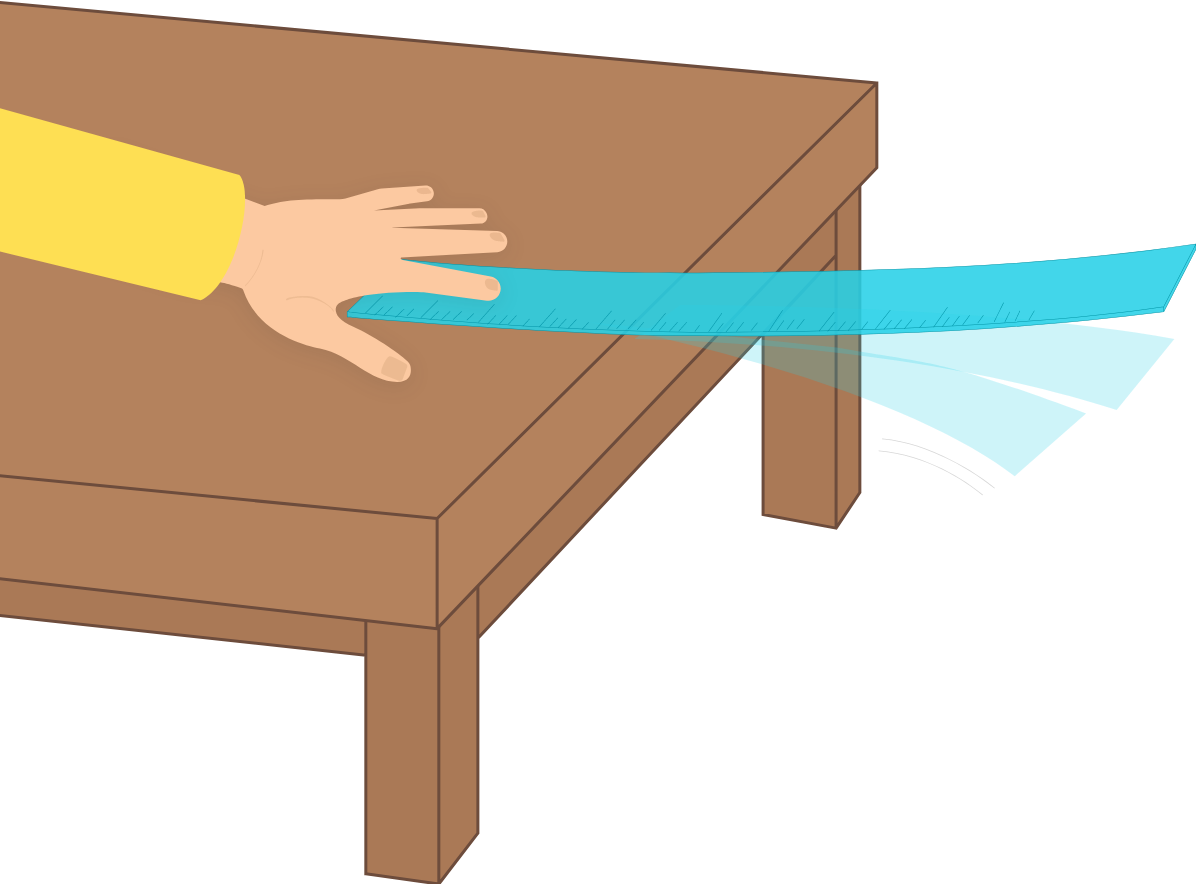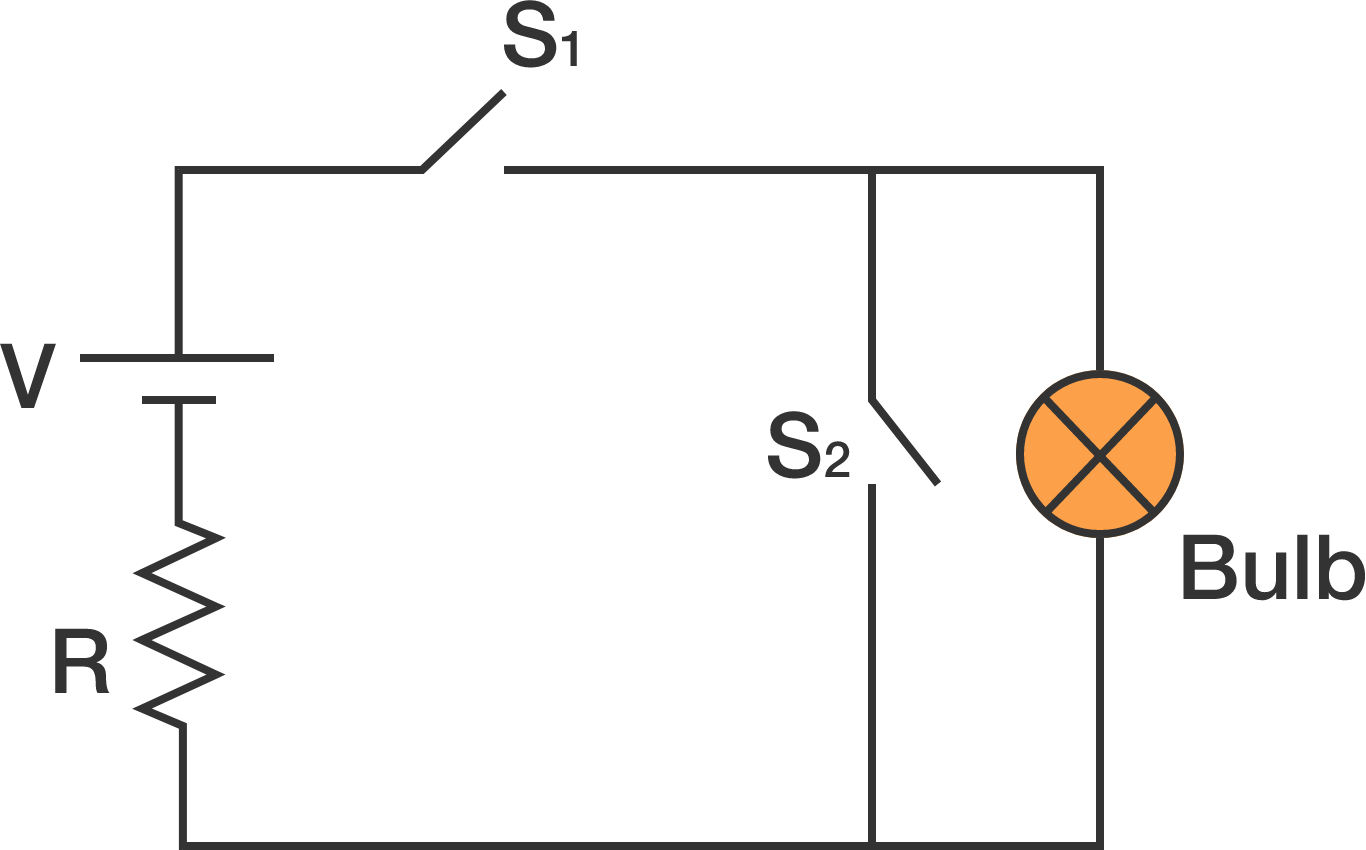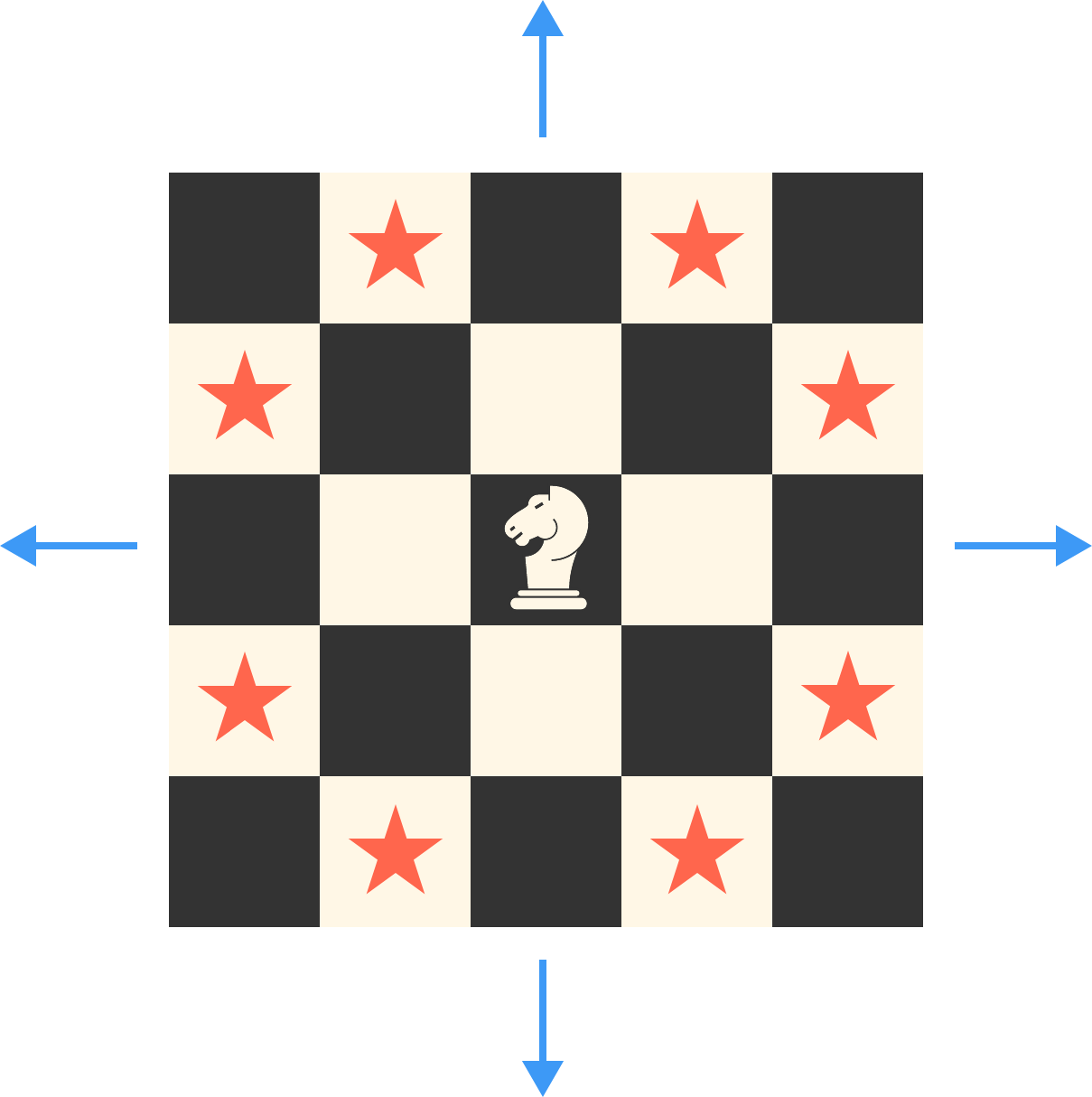# Problems of the Week

Contribute a problem

# 2017-09-04 BasicNed is trying to play music using a steel ruler extending beyond the table edge. When he strikes the ruler, a twang sound is produced.

If he wants to produce a higher frequency note, then what should he do?A pen costs an integer number of cents. There are 100 cents in a dollar.

9 of these pens cost between $11 and$12.
13 of these pens cost between $15 and$16.

What is the price of one pen in cents?A filament bulb is connected with a battery, a resistor, and two switches $S_1$ and $S_2,$ as shown in the diagram above. For which combination of the switches will the bulb glow?

Assume the connecting wires have negligible resistance.

$\begin{array} { r c r } 3^3 &=& {\color{#D61F06}{2}}7 \\ 3^4 &=& {\color{#D61F06}{8}}1 \\ 3^5 &=& 2{\color{#D61F06}{4}}3\\ 3^6 &=& 7{\color{#D61F06}{2}}9\\ 3^7 &=& 21{\color{#D61F06}{8}}7 \\ 3^8 &=& 65{\color{#D61F06}{6}}1 \end{array}$

The above shows the first few powers of 3, starting from $3^3$. Is it true that the second last digit (from the right) of $3^n$ for integers $n\,(> 2)$ is always an even number?There is a knight on the bottom left square of a $101\times 101$ chess board. What is the minimum number of moves required for the knight to get to the top right corner?


Note: A knight's valid moves are shown by the stars in the picture to the right.

×